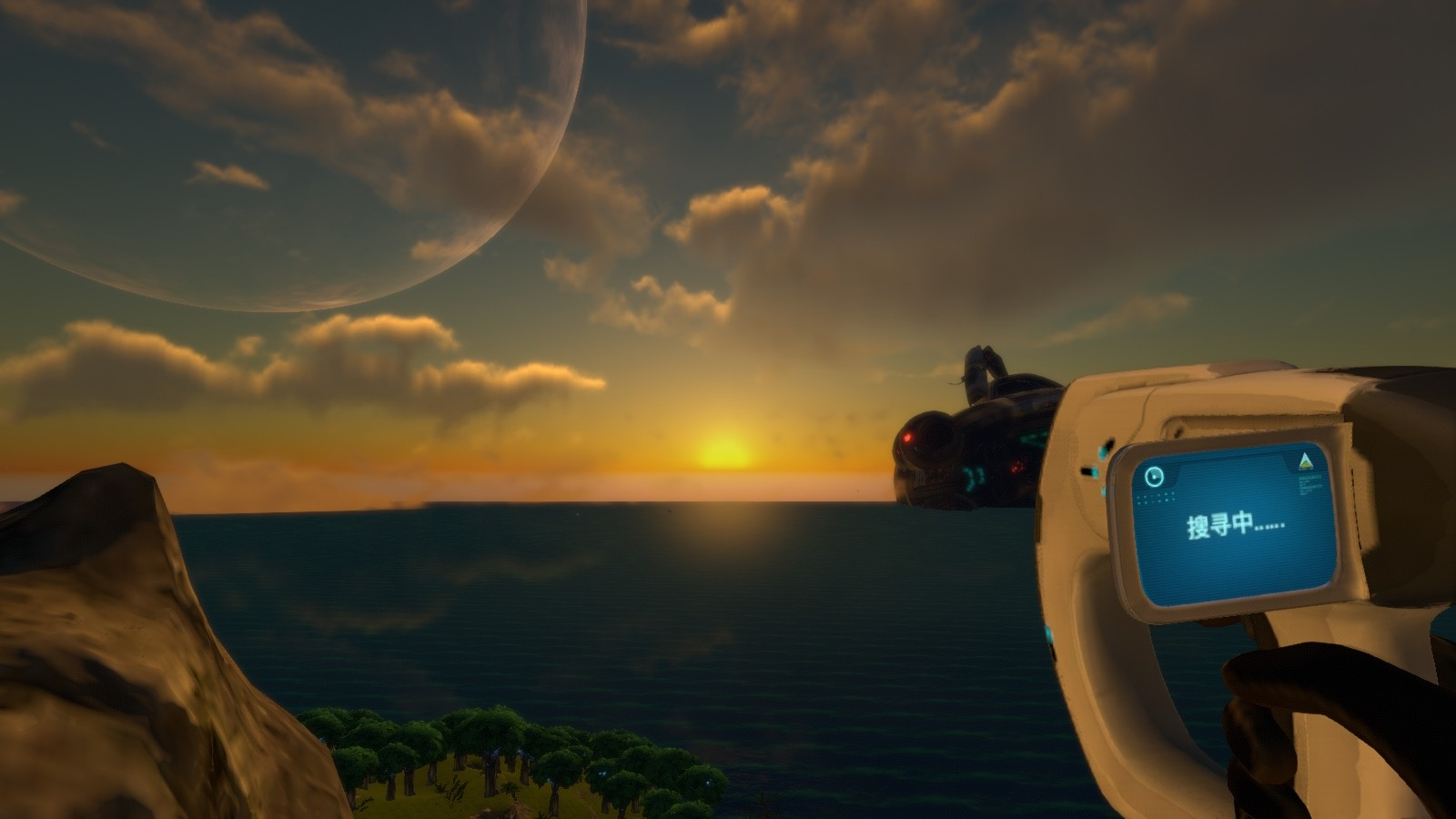#### 一代物品key合集

[本贴已启用专属短网址：blzx.cloud/snkey]

f3 f8同时打开，勾选掉禁用控制台选项，按~打开控制台然后输入spawn ID 或者itemID

1 = 石英

2 = 废料

3 = 纤维布

4 = 石灰岩 【钛.铜】

7 = 铜矿石

8 = 铅

9 = 盐块

12= 汞矿石

15= 玻璃

16= 钛

17= 硅胶

21= 金块

22= 镁

23= 结晶硫

27= 漂白剂

28= 银矿石

30= 钛锭

31= 沙石【铅.金.银】

32= 铜线

33= 接线盒

34= 高级接线盒

35= 洞穴硫磺

36= 钻石

37= 玄武岩【锂.镍】

38= 页岩【钻石.锂.金】

39= 黑曜石岩【钻石】

40= 锂

41= 塑钢锭

42= 陶瓷玻璃

43= 动力电池

44= 只能芯片

51= 铀

52= 氧化铝晶体

53= 盐酸

54= 磁铁矿

55= 聚笨胺

57= 人造纤维

59= 气凝胶

61= 笨

62= 润滑剂

63= 铀矿晶体

64= 反应堆控制棒

65= 用完的反应堆控制棒

66= 离子晶体

67= 离子晶体矩阵

68= 蓝晶石

69= 镍矿

70= 盐块【装甲】

71= 石英【装甲】

72= 铜【装甲】

73= 钛【装甲】

74= 铅【装甲】

75= 银【装甲】

76= 钻石【装甲】

77= 金块【装甲】

78= 磁铁【装甲】

79= 锂【装甲】

80= 汞【装甲】

81= 铀矿晶体【装甲】

82= 氧化铝晶体【装甲】

83= 镍矿【装甲】

2000= 海蛾号

2001= 海虾号

2005= 信号（不懂）

2100= 海蛾号深探模块

2101= 通用引擎效率模组

2102= 海蛾号太阳能充电器模组

2103= 通用存储升级模组

2104= 海蛾号电子防御模版

2105= 海蛾号船体加固模版

2109= 海蛾号鱼雷模块

2110= 海蛾号声纳模版

2111= 漩涡鱼雷

2112= 海蛾号MK1压力补偿模版

2113= 海蛾号MK2压力补偿模版

2114= 海蛾号MK3压力补偿模版

2115= 海虾喷射跳跃模块

2116= 海虾号电钻手臂

2117= 海虾热反应堆模版

2119= 毒气鱼雷

2120=海虾冲击炮手臂

2121= 海虾格斗手臂

2122= 海虾鱼雷手臂

2123= 海虾电钻手臂[扫描]

2124= 海虾冲击炮手臂[扫描]

2125= 海虾格斗手臂[扫描]

2126= 海虾鱼雷手臂[扫描]

2128= 海虾号初级深潜模块

2129= 海虾号高级深潜模块

3001= 树卵

3002= 海蛭

3003= 蓝色藤壶

3004= 蓝色藤壶簇

3010= 聚凝胶

3015 -- 3018= 小中大巨型库什

3019= 脑膜树

3020= 紫扇草

3021= 酸性蘑菇

3022= 触手草

3025= 珊瑚板

3026= 小风扇种子

3028 = 大珊瑚板

3029 = 树状蘑菇

3034 = 血油

3035 = 白蘑菇

3036 = 血油树[横]

3037 =血油树[竖]

3039 = 草蘑菇

3040 = 紫铃

3041 = 面包树

3042 = 紫颈瓶种子

3045 = 果子树

3046 = 紫薯树

3047 = 甜瓜

3050 = 皇冠草

3052 = 邪目草

3053 = 鬼手草

3054 = 紫罗兰

3055 = 红篮草

3056 = 红灌草

3057 = 红锥草

3058 = 贝壳草

3059 = 荧根草

3060 = 培根草

3061 = 海英草

3062 = 蛇菇

3063 = 外星生物的粪便

3064 = 宝石珊瑚

3065 = 水雷

3501 = 宝石盘碎片

3502 = 珊瑚管样本

3503 = 库什样本

3504 = 潜行者的牙齿

3506 = 面包树块

3507 = 橘子菇孢子

3508 = 紫颈瓶种子

3509 = 紫蘑菇孢子

3510 = 白蘑菇孢子

3511 = 草莓菇孢子

3512 = 紫铃孢子

3513 = 挂果树的果实

3514 = 紫薯

3515 = 小甜瓜

3516 = 甜瓜

3517 = 甜瓜种子

3518 = 脑珊瑚样本

3519 = 虎皮植物种子

3521 = 紫扇草种子

3522 = 小风扇种子

3523 = 触手草种子

3524 = 凝胶球孢子

3525 = 加布之羽种子

3526 = 皇冠草种子

3527 = 脑膜树种子

3528 = 体素花种子

3529 = 芭蕉草种子

3530 = 海葵种子

3531 = 邪目草果实

3532 = 鬼手草种子

3533 = 紫罗兰种子

3534 = 红篮草种子

3535 = 红灌草种子

3536 = 红锥草种子

3537 = 贝壳草种子

3538 = 荧根草种子

3539 = 培根草种子

3540 = 海樱花种子

3541 = 蛇菇孢子

4002 = 脑珊瑚

4004 = 虎皮草[攻击]

4006 = 失落之河植物很大

4200 = 红色先驱者芯片

4201 = 蓝色先驱者芯片

4202 = 橙色先驱者芯片

4203 = 白色先驱者芯片

4204 = 紫色先驱者芯片

4209 = 离子动力单元

4210 = 离子电池

4500 = 过滤净水

4501 = 未污染的水

4502 = 烤熟的大眼鱼

4503 = 烤熟的洞洞鱼

4504 = 烤熟的咖喱鱼

4505 = 烤熟的雷纳鱼

4506 = 烤熟的泡泡鱼

4507 = 烤熟的洗盘鱼

4508 = 烤熟的铁锹鱼

4509 = 烤熟的飞镖鱼

4510 = 烤熟的小眼仔

4511 = 烤熟的鬼眼鱼

4512 = 烤熟的圈圈鱼

4513 = 速食饼干

4514 = 急救包

4515 = 再生水

4516 = 大瓶过滤水

4517 = 烤熟的刺头鱼

4518 = 烤熟的岩浆小眼仔

4519 = 烤熟的岩浆飞镖鱼

4520 -- 4522 = 奶粉?[模型]

4523 = 纯正咖啡

4600 -- 4613 = 腌制

5900 = 火箭发射平台

6005 = 物竞天择海报2

SUB CYCLOPS

NODAMAGE

NOCOST

OXYGEN

NITROGEN(氮气)

BIOME [NAME]
.如果只输入BIOME，你会看到你所处BIOME的名称，与MC的F3类似。

WARP [X] [Y] [Z]

BATCH [X] [Y] [Z]

WARPME

KILL